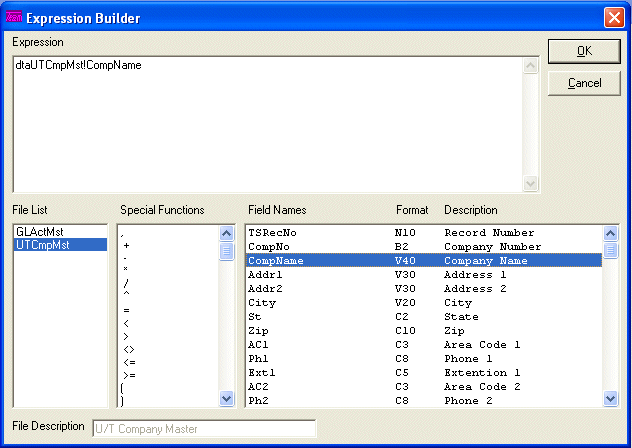R/G Expression Builder

This option is used create expressions that will be used in If Conditions, R/G Fields, Link Lists, and other various areas.

After selecting this option, the computer will display the R/G Expression Builder screen as follows:Expression ñ Enter a valid Expression to be used in an equation or report.  (When first entering this screen, the cursor will be positioned at the end of the Expression.)  You may also fill this Expression automatically by clicking and/or double clicking the File List, Special Functions, and Field List list boxes.  Click on Expressions Explanations And Examples to see how to setup a valid Expression.

File List ñ The File List box allows you to click a File that you wish to use. After clicking a File, the computer will display the File Description and automatically fill the Field List and Field Description List with all the fields from the selected File

Special Functions ñ The Special Functions list box allows you to insert a Special Function into the Expression by double-clicking it from the list.

Field List ñ This list contains Field Names, Field Formats, and Field Descriptions for the selected File.  See Insert Field Name to find out the easiest way to insert a valid field name into an expression.

The following are examples of  the Field Formats and their usage.

B7 - Blank Filled - This is a string variable and can contain numbers, and spaces.  The size of this type should always be the same and it is identified by the number following the B in this example the maximum size is 7.  The fields that have this type will contain an appropriate quantity of spaces before the number, so that the length of this field always equals the maximum size.  (i.e. A B7 type that has a value of 100 would be the same as "    100" (4 spaces followed by the 3 digit number which makes a total of 7 characters).)

C10 - Capital Letters Only  - This is a string variable and can contain capital letters, numbers, and special characters.  The maximum size of this type is identified by the number following the C in this example the maximum size is 10.

D8 - Date  - This is a string variable and contains a date with the format of "YYYYMMDD" where YYYY is the four-digit year, MM is the two-digit month and DD is the two-digit day.  (i.e. the date December 31, 2009 would be stored as "20091231".)

M - Memo - This is a string variable and can contain letters, numbers, and special characters.  The size of this type is unlimited.

N5 - Number (No Decimal) - This is a numeric variable and can contain numbers, plus sign, and minus sign.  The maximum size of this type is identified by the number following the N in this example the maximum size is 5.  This type will only store whole numbers.

N10.2 - Number - This is a numeric variable and can contain numbers, plus sign, minus sign, and a period.  The maximum size of this type is identified by the number following the N in this example the maximum size is 10 numbers to the left of the decimal and 2 numbers to the right of the decimal.

T8 - Time  - This is a string variable and contains a time with the format of "HH:MM:SS" where HH is a 2-digit hour (expressed as military time), MM is a two-digit minute and SS is a two-digit second.  (i.e. the date 1:05:15 p.m. would be stored as "13:05:15".)

V30 - Variety Text - This is a string variable and can contain letters, numbers, and special characters.  The maximum size of this type is identified by the number following the V in this example the maximum size is 30.

X1 - Yes/No - This is a string variable and will contain a "1" (which means Yes) or "0" (which means No).  The size of this type is one.

Once the expression is built, click the Ok button to accept the changes or Cancel button to reject the changes.

No Right Mouse